STUDENT
TEACHER

Surface Area of Cylinders Calculator

STUDENT
TEACHER

Surface Area of Cylinders Calculator

# Surface Area of Cylinders

CALCULATOR

## Surface Area of Cylinders Calculator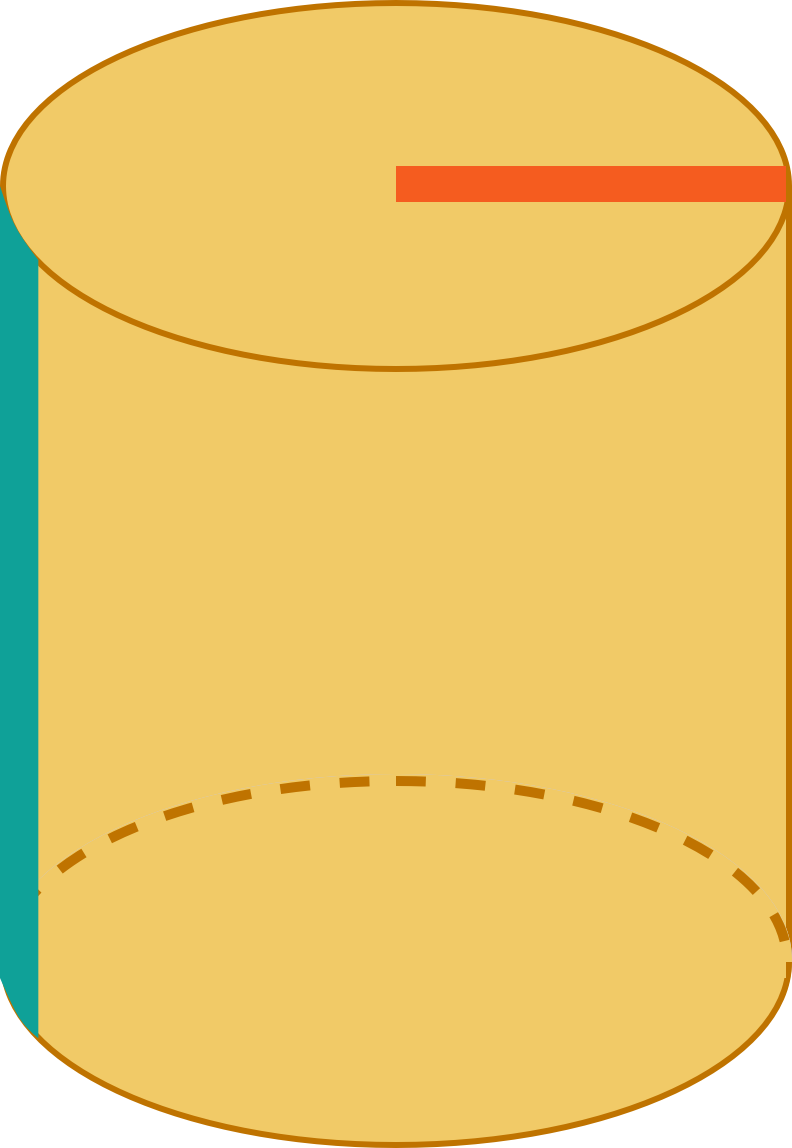We have some questions for you! Help us out through this
INTRO
The surface area of a 3D shape is equal to the sum of the areas of all the faces (sides) of that shape. A helpful way to think about it is:
surface area = sum of face areas
We can also think about surface area in terms of gifts! The surface area is like the minimum amount of wrapping paper we need to prep a gift for its surprise reveal. 🤩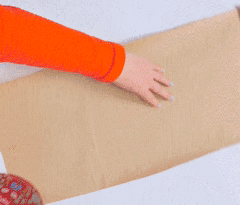If we were to unwrap a gift, the amount of wrapping would be equal to the surface area of the object it was covering.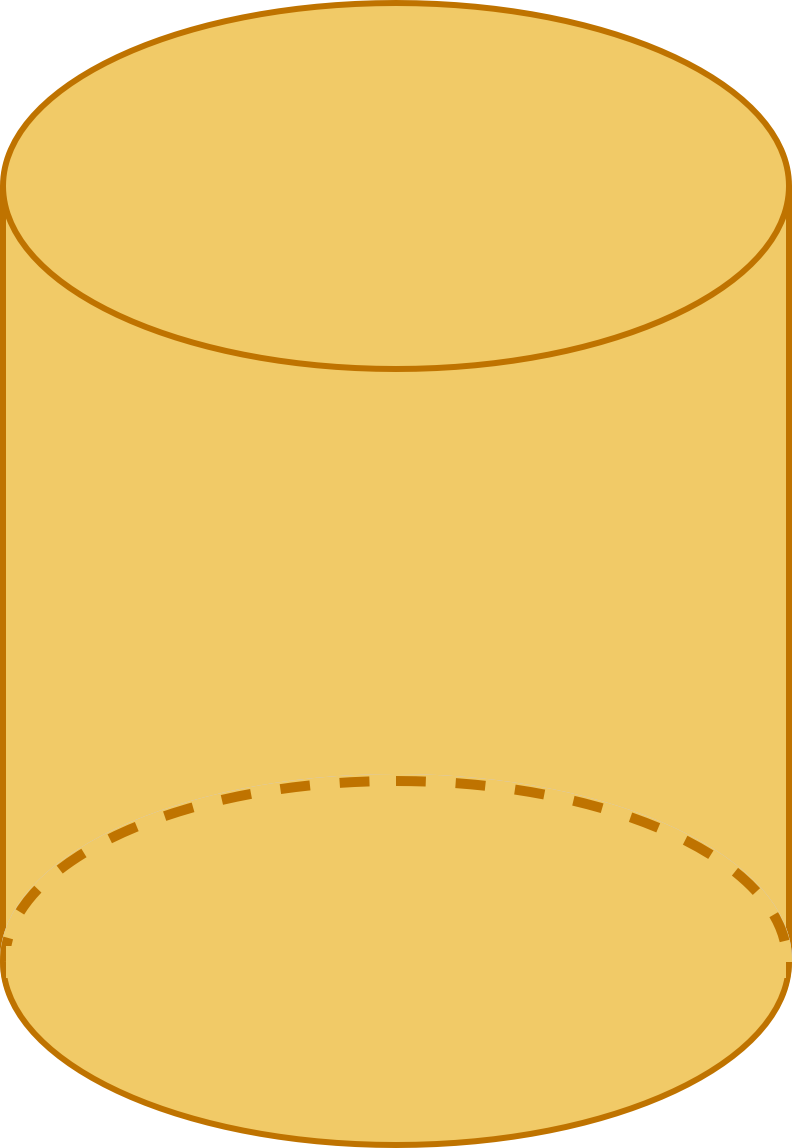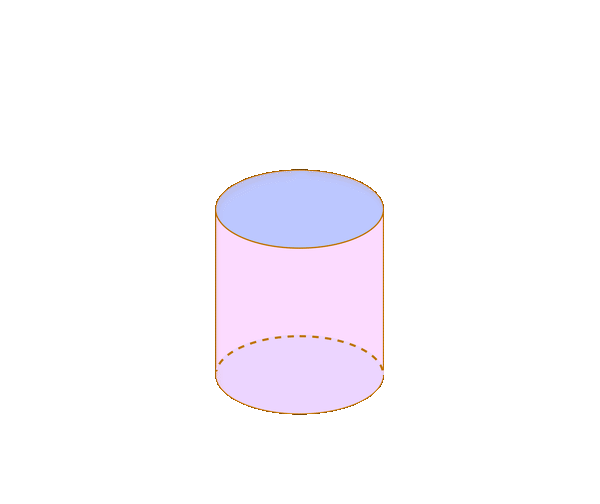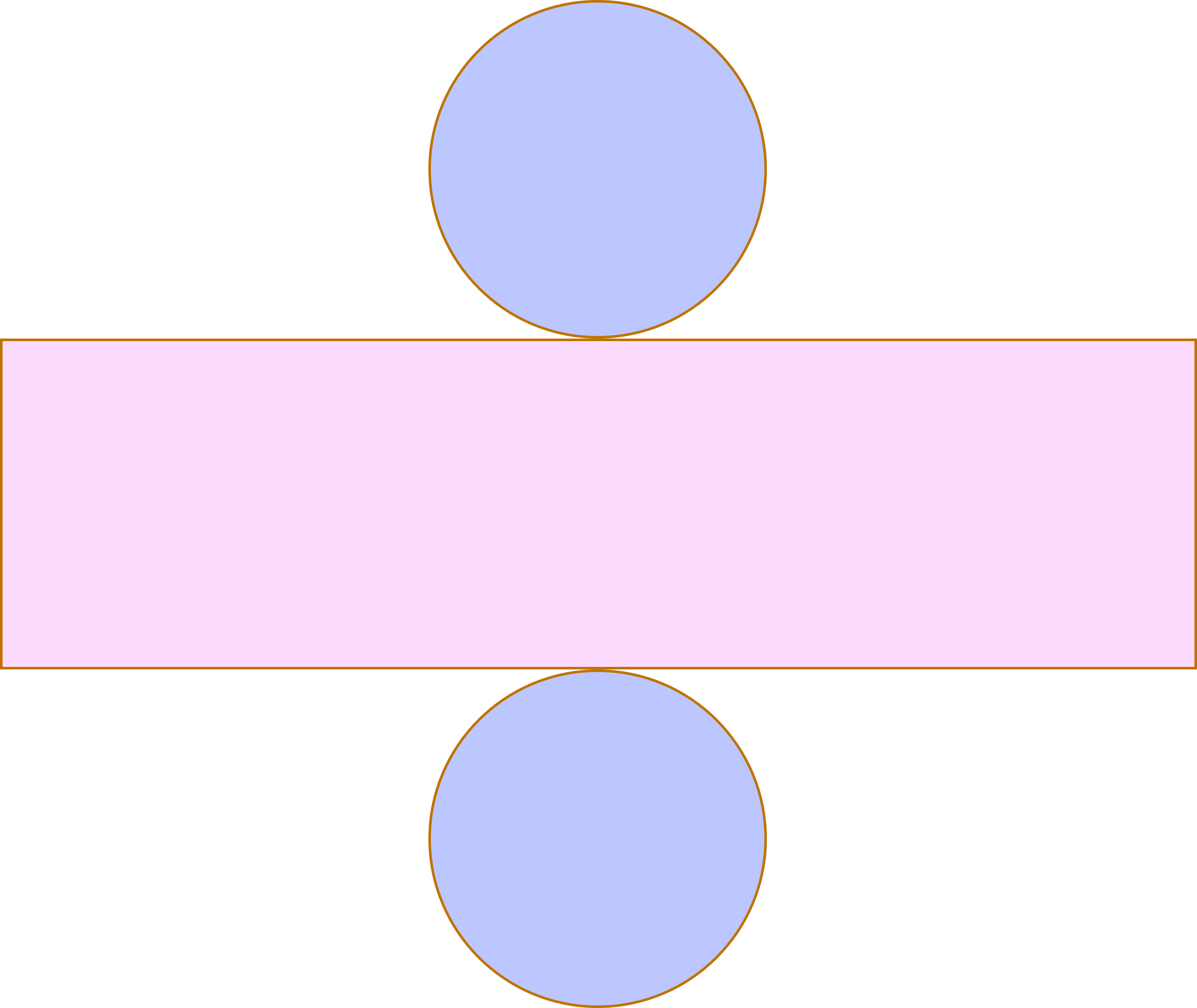When we “unwrap” a 3D shape, we get what we call a net. A surface area net shows us the different 2D shapes that make up the faces of the 3D shape.
We can then find the area of each face and then add them all together to find the total surface area.

### What is lateral surface area?

When working with pyramids, cylinders, and cones, you may also be asked to find the lateral surface area.
Lateral surface area is simply the total surface area of an object minus the area of its base(s).
Check out our
Calculator
or explore our
Lesson
and
Practice

KEY STEPS

## How to Find Surface Area of Cylinders

### Step 1. Unwrap the cylinder.### Step 2. Calculate the area of each part of the net that makes up the cylinder.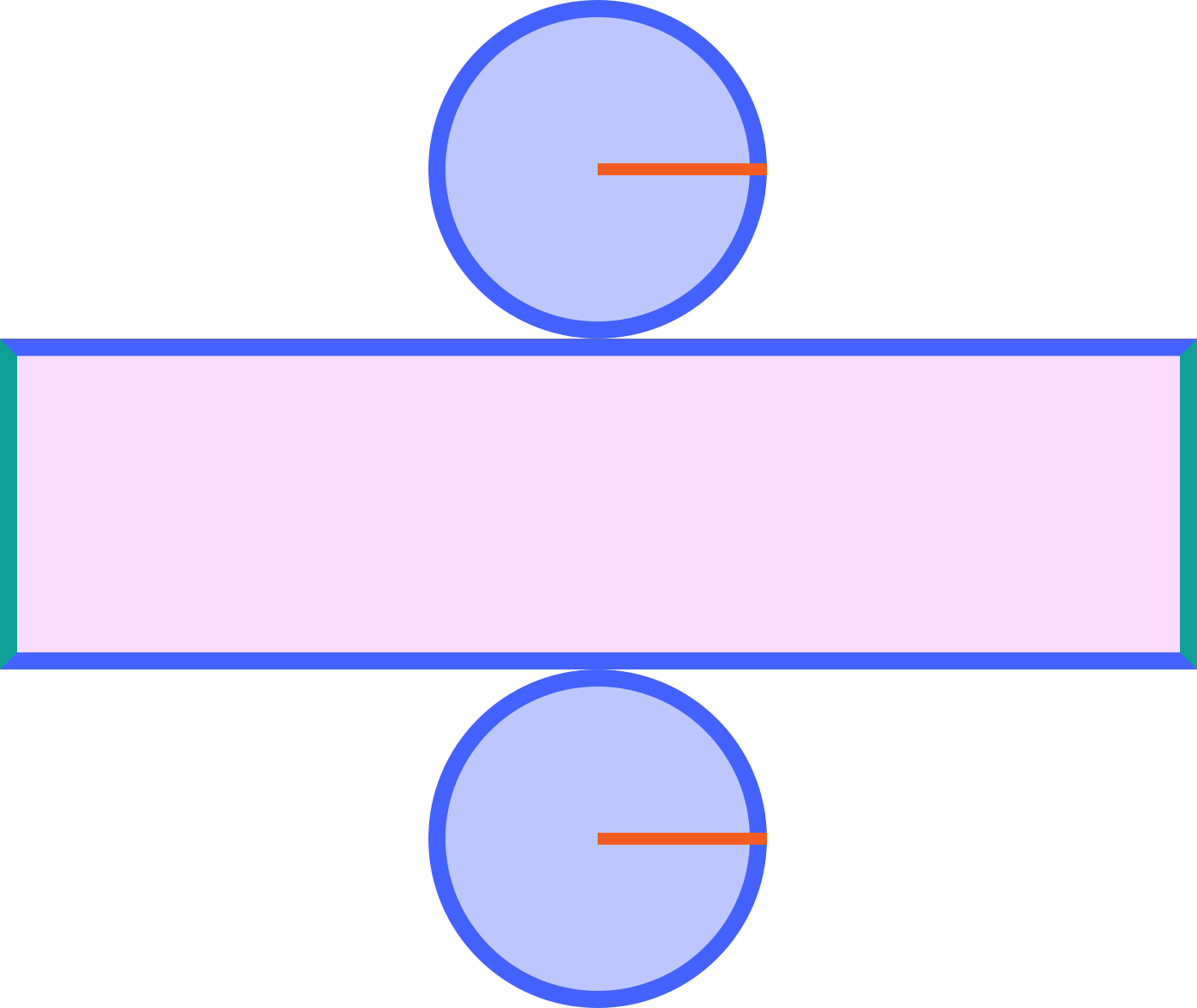Rectangle Circles

### Step 3. Add up the areas of the shapes that make up the cylinder.

LESSON
Surface Area of Cylinders
PRACTICE
Surface Area of Cylinders
CONCLUSION
Leave Feedback